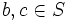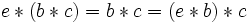# Left-associative elements of magma form submagma

## Statement

Let$(S,*)$ be a magma (a set$S$ with binary operation$*$). Call an element$a \in S$ a left-associative element (or left nuclear element) if the following holds:$a * (b * c) = (a * b) * c \ \forall \ b,c \in S$

Then:

1. The set of left-associative elements of$S$ forms a submagma of$S$. Further, this submagma is a semigroup, since it is associative. This submagma is termed the left nucleus of the magma.
2. If$S$ contains a left neutral element$e$, then$e$ is left-associative, and is a left neutral element for the submagma of left-associative elements.
3. If$S$ contains a left nil$n$, then$n$ is left-associative.

## Related facts

### Associativity in other positions

All these proofs make crucial use of the associativity pentagon: the pentagon describing the relation between the five different ways of associating a product of length four.

## Proof

### Proof idea

The idea behind the proof is the associativity pentagon, which states that the five different ways of parenthesizing an expression of length four form a cyclic pentagon, with each expression related to exactly two others via a single application of the associativity law. In order to move along one edge of the pentagon, we instead move along the path comprising the remaining four edges, and use the fact that each step there is allowed because one of$a_1$ and$a_2$ is the left term in each of the applications of the law.

### Proof of (1)

Given: A magma$(S,*)$, two left-associative elements (i.e., left nuclear)$a_1,a_2 \in S$

To prove:$a_1 * a_2$ is left-associative (i.e., left nuclear)

Proof: We need to show that, for any$b, c \in S$, we have:$((a_1 * a_2) * b) * c = (a_1 * a_2) * (b * c)$

Let's do this. Start with the left side and proceed as follows:$((a_1 * a_2) * b) * c = (a_1 * (a_2 * b)) * c = a_1 * ((a_2 * b) * c) = a_1 * (a_2 * (b * c)) = (a_1 * a_2) * (b * c)$

In three of the steps, we use the fact that$a_1$ is left-associative, and in one of the steps, we use the fact that$a_2$ is left-associative.

Here is a diagrammatic depiction of the proof:

### Proof of (2)

Given: A magma$(S,*)$ with left neutral element$e$.

To prove:$e$ is in the submagma of left-associative elements and is left neutral for the submagma.

Proof: For any$b,c \in S$,$e * (b * c) = b * c = (e * b) * c$, so$e$ is left-associative. Since$e * b = b$ for all$b \in S$,$e * b = b$ for all left-associative elements in particular.

### Proof of (3)

Given: A magma$(S,*)$ with left nil$n$.

To prove:$n$ is in the submagma of left-associative elements and is a left nil for that submagma.

Proof: For any$b,c \in S$,$n * (b * c) = n = n * c = (n * b) * c$, so$n$ is left-associative. Since$n * b = n$ for all$b \in S$,$n * b = n$ for all left-associative elements in particular.